• 在单图像去模糊中，传统的基于优化的方法和最近的基于神经网络的方法都非常成功，即在金字塔中逐步恢复不同分辨率的锐利图像。在本文中，我们研究了这种策略，并提出了一个规模递归网络（SRN-DeblurNet）来完成这项...
• 图像去模糊，各种传统滤波算法，逆滤波、维纳滤波、LR算法等等。。。针对图像运动模糊领域
• Keras implementation of "DeblurGAN: Blind Motion Deblurring Using Conditional Adversarial Networks
• 雷锋网 (公众号：雷锋网) 按：本文为 雷锋字幕组 编译的技术博客，原标题GAN with Keras: Application to Image Deblurring，作者为Raphaël ...这篇文章主要介绍在 Keras 中 搭建GAN实现图像去模糊 。所有的Ker...

雷锋网 (公众号：雷锋网) 按：本文为 雷锋字幕组 编译的技术博客，原标题GAN with Keras: Application to Image Deblurring，作者为Raphaël Meudec。

翻译 | 廖颖  陈俊雅  整理 | 凡江2014年 Ian Goodfellow 提出了 生成对抗网络(GAN) 。这篇文章主要介绍在 Keras 中 搭建GAN实现图像去模糊 。所有的Keras代码可点击 这里 。

快速回忆生成对抗网络

GAN中两个网络的训练相互竞争。生成器( generator) 合成具有说服力的假输入 来误导判别器(discriminator )，而判别器则是来 识别这个输入是真的还是假的 。生成对抗网络训练过程— 来源

训练过程主要有三步

根据噪声，生成器 合成假的输入

用真的输入和假的输入 共同训练判别器

训练整个模型：整个模型中判别器与生成器连接

注意：在第三步中，判别器的权重是固定的

将这两个网络连接起来是由于生成器的输出没有可用的反馈。 我们唯一的准则就是看判别器是否接受生成器的合成的例子。

这些只是对生成对抗网络的一个简单回顾，如果还是不够明白的话，可以参考 完整介绍 。

数据

Ian Goodfellow首次使用GAN模型是生成MNIST数据。 而本篇文章是 使用生成对抗网络进行图像去模糊 。因此生成器的输入不是噪声，而是模糊图像。

数据集来自 GOPRO数据 ，你可以下载 精简版数据集 (9GB)，也可以下载 完整版数据集 (35GB)。其中 包含了来自不同街道视角的人造模糊图像 ，根据不同的场景将数据集分在各个子文件夹中。

我们先把图像分到 A (模糊)和 B (清晰)两个文件夹。这个 A&B 结构对应于原始文章 pix2pix 。我创建了一个 自定义脚本来执行这个任务。 看看 README 后尝试一下吧。

模型

训练过程还是一样，首先来看一下神经网络结构。

生成器

生成器要生成清晰图像，网络是基于 ResNet blocks 的，它可以记录对原始模糊图像操作的过程。原文还使用了基于 UNet 的版本，但我目前还没有实现。这两种结构都可以很好地进行图像去模糊。DeblurGAN 生成器网络 结构 — 来源

核心是采用 9 个ResNet blocks 对原始图像进行上采样。来看一下Keras上的实现！ResNet 层就是一个基本的卷积层，其中,输入和输出相加，形成最终输出。生成器结构的 Keras 实现

按照计划，用9个ResNet blocks对输入进行上采样。我们 在输入到输出增加一个连接 ，然后除以2 来对输出进行归一化。

这就是生成器了! 我们再来看看判别器的结构吧。

判别器

判别器的目标就是要确定一张输入图片是否为合成的。因此判别器的结构采用卷积结构，而且是一个 单值输出 。判别器结构的 Keras 实现

最后一步就是建立完整的模型。 这个GAN的一个特点 就是输入的是真实图片而不是噪声 。因此我们就有了 一个对生成器输出的直接反馈 。接下来看看采用两个损失如何充分利用这个特殊性。

训练

损失

我们提取生成器最后和整个模型最后的损失。

第一个是 感知损失 ，根据生成器输出直接可以计算得到。第一个损失保证 GAN 模型针对的是去模糊任务。 它比较了VGG第一次卷积的输出 。第二个损失是对整个模型输出计算的 Wasserstein loss ， 计算了两张图像的平均差值 。众所周知，这种损失可以提高生成对抗网络的收敛性。训练流程然后我们进行epochs(一个完整的数据集通过了神经网络一次并且返回了一次的过程，称为一个epoch)，并将整个数据集分批次(batches)。最后根据两者的损失，可以相继训练判别器和生成器。用生成器生成假的输入，训练判别器区别真假输入，并对整个模型进行训练。你可以参考 Github 来查看完整的循环。

实验

我使用的是在 AWS 实例(p2.xlarge)上配置深度学习 AMI (version 3.0)进行的 。对 GOPRO 精简版 数据集的训练时间大约有 5 个小时(50个epochs)。

图像去模糊结果从左到右：原始图像，模糊图像，GAN 输出

上面的输出结果都是我们用 Keras 进行 Deblur GAN 的结果。即使是对高度模糊，网络也可以减小模糊，产生一张具有更多信息的图片，使得车灯更加汇聚，树枝更加清晰。左图： GOPRO 测试图像，右图：GAN 输出结果

因为引入了VGG来计算损失，所以会产生图像顶部出现感应特征的局限。左图： GOPRO 测试图像，右图：GAN 输出结果

希望你们可以喜欢这篇关于生成对抗网络用于图像去模糊的文章。 你可以评论，关注我或者 联系我 。

如果你对机器视觉感兴趣，我们还写过一篇用Keras实现 基于内容的图像复原 。下面是生成对抗网络资源的列表。左图： GOPRO 测试图像，右图：GAN 输出结果

生成对抗网络资源

更多文章，关注雷锋网 雷锋网雷锋网

添加雷锋字幕组微信号(leiphonefansub)为好友

备注「我要加入」，To be an  AI  Volunteer ！雷锋网原创文章，未经授权禁止转载。详情见 转载须知 。展开全文• 这是一个基于python图像分割的模糊c均值算法
• 对于运动引起的图像模糊，最简单的方法是直接做逆滤波，但是逆滤波对加性噪声特别敏感，使得恢复的图像几乎不可用。最小均方差（维纳）滤波用来去除含有噪声的模糊图像，其目标是找到未污染图像的一个估计，使它们...

一、维纳滤波

对于运动引起的图像模糊，最简单的方法是直接做逆滤波，但是逆滤波对加性噪声特别敏感，使得恢复的图像几乎不可用。最小均方差（维纳）滤波用来去除含有噪声的模糊图像，其目标是找到未污染图像的一个估计，使它们之间的均方差最小，可以去除噪声，同时清晰化模糊图像。
y ( t ) = h ( t ) ⨂ x ( t ) + n ( t ) y(t)=h(t)\bigotimes x(t)+n(t)

其中：
⨂ \bigotimes 是卷积符号
x ( t ) x(t) 是在时间 t t 刻输入的信号（未知）
h ( t ) h(t) 是一个线性时间不变系统的脉冲响应（已知）
n ( t ) n(t) 是加性噪声，与 x ( t ) x(t) 不相关（未知）
y ( t ) y(t) 是我们观察到的信号
我们的目标是找出这样的卷积函数 g ( t ) g(t) ，这样我们可以如下得到估计的 x ( t ) x(t)
x ^ ( t ) = g ( t ) ∗ y ( t ) \hat{x}(t)=g(t)∗y(t)

这里 x ^ ( t ) \hat{x}(t) x ( t ) x(t) 的最小均方差估计。
基于这种误差度量， 滤波器可以在频率域如下描述
G ( f ) = H ∗ ( f ) S ( f ) ∣ H ( f ) ∣ 2 S ( f ) + N ( f ) = H ∗ ( f ) ∣ H ( f ) ∣ 2 + N ( f ) / S ( f ) G(f)=\frac{H^∗(f)S(f)}{|H(f)|^2S(f)+N(f)}=\frac{H^∗(f)}{|H(f)|^2+N(f)/S(f)}

这里：
G ( f ) G(f) )和 H ( f ) H(f) g g h h 在频率域ff的傅里叶变换。
S ( f ) S(f) 是输入信号 x ( t ) x(t) 的功率谱。
N ( f ) N(f) 是噪声的 n ( t ) n(t) 的功率谱。
上标 ∗ ^∗ 代表复数共轭。
滤波过程可以在频率域完成： X ^ ( f ) = G ( f ) ∗ Y ( f ) \hat{X}(f)=G(f)∗Y(f)

这里 X ^ ( f ) \hat{X}(f) x ^ ( t ) \hat{x}(t) 的傅里叶变换，通过逆傅里叶变化可以得到去卷积后的结果 x ^ ( t ) \hat{x}(t)

上面的式子可以改写成更为清晰的形式：
G ( f ) = 1 H ( f ) [ ∣ H ( f ) ∣ 2 ∣ H ( f ) ∣ 2 + N ( f ) S ( f ) ] = 1 H ( f ) [ ∣ H ( f ) ∣ 2 ∣ H ( f ) ∣ 2 + 1 S N R ( f ) ] G(f) = \frac{1}{H(f)}\left [ \frac{|H(f)|^2}{|H(f)|^2 + \frac{N(f)}{S(f)}} \right ] = \frac{1}{H(f)}\left [ \frac{|H(f)|^2}{|H(f)|^2 + \frac{1}{SNR(f)}} \right ]

这里 H ( f ) H(f) h h 在频率域 f f 的傅里叶变换。 S N R ( f ) = S ( f ) / N ( f ) SNR(f)=S(f)/N(f) 是信号噪声比。当噪声为零时（即信噪比趋近于无穷），方括号内各项也就等于1，意味着此时刻维纳滤波也就简化成逆滤波过程。但是当噪声增加时，信噪比降低，方括号里面值也跟着降低。这说明，维纳滤波的带通频率依赖于信噪比。

代码示例：如下代码参考于【博主】，自己解决了对RGB图片的支持，与诸位共勉。

import matplotlib.pyplot as plt
import numpy as np
from numpy import fft
import math
import cv2

# 仿真运动模糊
def motion_process(image_size, motion_angle):
PSF = np.zeros(image_size)
print(image_size)
center_position = (image_size - 1) / 2
print(center_position)

slope_tan = math.tan(motion_angle * math.pi / 180)
slope_cot = 1 / slope_tan
if slope_tan <= 1:
for i in range(15):
offset = round(i * slope_tan)  # ((center_position-i)*slope_tan)
PSF[int(center_position + offset), int(center_position - offset)] = 1
return PSF / PSF.sum()             # 对点扩散函数进行归一化亮度
else:
for i in range(15):
offset = round(i * slope_cot)
PSF[int(center_position - offset), int(center_position + offset)] = 1
return PSF / PSF.sum()

# 对图片进行运动模糊
def make_blurred(input, PSF, eps):
input_fft = fft.fft2(input)             # 进行二维数组的傅里叶变换
PSF_fft = fft.fft2(PSF) + eps
blurred = fft.ifft2(input_fft * PSF_fft)
blurred = np.abs(fft.fftshift(blurred))
return blurred

def inverse(input, PSF, eps):                # 逆滤波
input_fft = fft.fft2(input)
PSF_fft = fft.fft2(PSF) + eps            # 噪声功率，这是已知的，考虑epsilon
result = fft.ifft2(input_fft / PSF_fft)  # 计算F(u,v)的傅里叶反变换
result = np.abs(fft.fftshift(result))
return result

def wiener(input, PSF, eps, K=0.01):        # 维纳滤波，K=0.01
input_fft = fft.fft2(input)
PSF_fft = fft.fft2(PSF) + eps
PSF_fft_1 = np.conj(PSF_fft) / (np.abs(PSF_fft) ** 2 + K)
result = fft.ifft2(input_fft * PSF_fft_1)
result = np.abs(fft.fftshift(result))
return result

def normal(array):
array = np.where(array < 0,  0, array)
array = np.where(array > 255, 255, array)
array = array.astype(np.int16)
return array

def main(gray):
channel = []
img_h, img_w = gray.shape[:2]
PSF = motion_process((img_h, img_w), 60)      # 进行运动模糊处理
blurred = np.abs(make_blurred(gray, PSF, 1e-3))

result_blurred = inverse(blurred, PSF, 1e-3)  # 逆滤波
result_wiener = wiener(blurred, PSF, 1e-3)    # 维纳滤波

blurred_noisy = blurred + 0.1 * blurred.std() * \
np.random.standard_normal(blurred.shape)  # 添加噪声,standard_normal产生随机的函数
inverse_mo2no = inverse(blurred_noisy, PSF, 0.1 + 1e-3)   # 对添加噪声的图像进行逆滤波
wiener_mo2no = wiener(blurred_noisy, PSF, 0.1 + 1e-3)     # 对添加噪声的图像进行维纳滤波
channel.append((normal(blurred),normal(result_blurred),normal(result_wiener),
normal(blurred_noisy),normal(inverse_mo2no),normal(wiener_mo2no)))
return channel

if __name__ == '__main__':
b_gray, g_gray, r_gray = cv2.split(image.copy())

Result = []
for gray in [b_gray, g_gray, r_gray]:
channel = main(gray)
Result.append(channel)
blurred = cv2.merge([Result, Result, Result])
result_blurred = cv2.merge([Result, Result, Result])
result_wiener = cv2.merge([Result, Result, Result])
blurred_noisy = cv2.merge([Result, Result, Result])
inverse_mo2no = cv2.merge([Result, Result, Result])
wiener_mo2no = cv2.merge([Result,  Result, Result])

#========= 可视化 ==========
plt.figure(1)
plt.xlabel("Original Image")
plt.imshow(np.flip(image, axis=2))                         # 显示原图像

plt.figure(2)
plt.figure(figsize=(8, 6.5))
imgNames = {"Motion blurred":blurred,
"inverse deblurred":result_blurred,
"wiener deblurred(k=0.01)":result_wiener,
"motion & noisy blurred":blurred_noisy,
"inverse_mo2no":inverse_mo2no,
'wiener_mo2no':wiener_mo2no}
for i,(key,imgName) in enumerate(imgNames.items()):
plt.subplot(231+i)
plt.xlabel(key)
plt.imshow(np.flip(imgName, axis=2))
plt.show()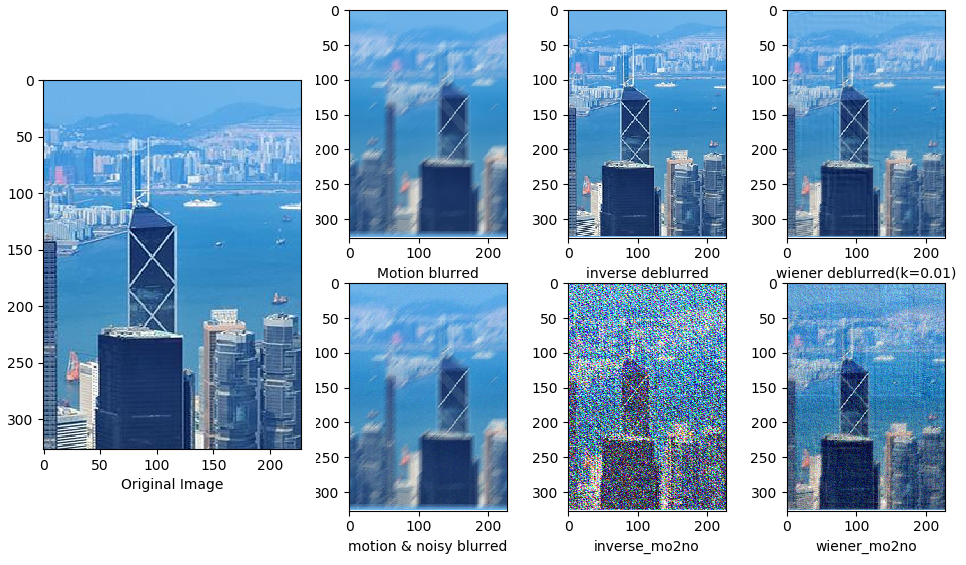二、约束最小二乘方滤波

约束最小二乘方滤波（Constrained Least Squares Filtering，aka Tikhonov filtration,Tikhonov regularization）核心是H对噪声的敏感性问题。减少噪声敏感新问题的一种方法是以平滑度量的最佳复原为基础的，建立下列约束条件：
C = ∑ 0 M − 1 ∑ 0 N − 1 [ ∇ 2 f ( x , y ) ] 2 C = \sum_0^{M-1}\sum_0^{N-1}[\nabla^2f(x,y)]^2

约束条件： ∣ ∣ G − H F ^ ∣ ∣ 2 2 = ∣ ∣ N ∣ ∣ 2 2 ||G-H\hat{F}||_2^2 = ||N||_2^2
这里， F ^ \hat{F} 是为退化图像的估计， N N 为加性噪声，拉普拉斯算子 ∇ 2 \nabla^2 在这里表示平滑程度。

推导
将上式表示成矩阵形式，同时将约束项转换成拉格朗日乘子项：
∣ ∣ P F ^ ∣ ∣ 2 2 − λ ( ∣ ∣ G − H F ^ ∣ ∣ 2 2 − ∣ ∣ N ∣ ∣ 2 2 ) ||P\hat{F}||_2^2 - \lambda \left ( ||G-H\hat{F}||_2^2 - ||N||_2^2 \right )

最小化上代价函数，对 F ^ \hat{F} 求导，令等零有： P ∗ P F ^ = λ H ∗ ( G − H F ^ ) P^∗P\hat{F}=λH^∗(G−H\hat{F})
最后可得到：
F ^ = λ H ∗ G λ H ∗ H + P ∗ P = [ H ∗ ∣ ∣ H ∣ ∣ 2 2 + λ ∣ ∣ P ∣ ∣ 2 2 ] G \hat{F} = \frac{ \lambda H^*G}{ \lambda H^*H + P^*P} = \left [ \frac{H^*}{||H||_2^2 + \lambda||P||_2^2 } \right ]G

P P 是函数
P = [ 0 − 1 0 − 1 4 − 1 0 − 1 0 ] P = \begin{bmatrix} 0 &amp; -1 &amp;0 \\ -1 &amp; 4 &amp;-1 \\ 0 &amp; -1 &amp; 0 \end{bmatrix}

三、psf2otf ,circShift

circshift(psf,K)

当K为一个数字时，只对矩阵进行上下平移，当K为一个坐标时，会对矩阵进行上下和左右两个方向进行平移。示例如下：
执行：平移坐标(-1,-1)，对矩阵进行上移，左移1个单位，效果如下:
[ 0 − 1 0 − 1 4 − 1 0 − 1 0 ] ⇒ [ 4 − 1 − 1 − 1 0 0 − 1 0 0 ] \begin{bmatrix} 0 &amp; -1 &amp; 0\\ -1 &amp; 4 &amp; -1\\ 0 &amp; -1 &amp; 0 \end{bmatrix} \Rightarrow \begin{bmatrix} 4 &amp; -1 &amp; -1\\ -1 &amp; 0 &amp; 0\\ -1 &amp; 0 &amp; 0 \end{bmatrix}

示例2：平移坐标(1,2)，对矩阵进行下移1个单位，右移2个单位，效果如下:
[ 1 2 3 4 5 6 7 8 9 10 11 12 13 14 15 16 17 18 19 20 ] ⇒ [ 19 20 16 17 18 4 5 1 2 3 9 10 6 7 8 14 15 11 12 13 ] \begin{bmatrix} 1&amp; 2&amp; 3&amp; 4&amp; 5\\ 6&amp; 7&amp; 8&amp; 9&amp; 10\\ 11&amp;12&amp;13&amp;14&amp;15\\ 16&amp;17&amp;18&amp;19&amp;20\\ \end{bmatrix} \Rightarrow \begin{bmatrix} 19&amp;20&amp;16&amp;17&amp;18\\ 4&amp;5&amp;1&amp;2&amp;3\\ 9&amp;10&amp;6&amp;7&amp;8\\ 14&amp;15&amp;11&amp;12&amp;13 \end{bmatrix}

psf2otf()

依次执行效果如下：
[ 0 − 1 0 − 1 4 − 1 0 − 1 0 ] ⇒ [ 0. + 0. j 3. + 0. j 3. + 0. j 3. + 0. j 6. + 0. j 6. + 0. j 3. + 0. j 6. + 0. j 6. + 0. j ] ⇒ [ 0 3 3 3 6 6 3 6 6 ] \begin{bmatrix} 0 &amp; -1 &amp; 0\\ -1 &amp; 4 &amp; -1\\ 0 &amp; -1 &amp; 0 \end{bmatrix} \Rightarrow \begin{bmatrix} 0.+0.j &amp; 3.+0.j &amp; 3.+0.j\\ 3.+0.j &amp; 6.+0.j &amp; 6.+0.j\\ 3.+0.j &amp; 6.+0.j &amp; 6.+0.j \end{bmatrix} \Rightarrow \begin{bmatrix} 0&amp; 3&amp; 3\\ 3 &amp; 6 &amp; 6\\ 3 &amp; 6 &amp; 6 \end{bmatrix}
其中 np.fft.fft2()返回值为复数，可以用np.real()获取复数的实部，np.imag()用来获取虚部。
代码示例：自己用numpy重写了matlab里面的psf2otf这个函数，
或者查看：https://blog.csdn.net/wsp_1138886114/article/details/97611270

# coding: utf-8
import numpy as np
import matplotlib.pyplot as plt
from numpy import fft
import cv2
from temp_004 import psf2otf

def motion_blur(gray, degree=7, angle=60):
gray = np.array(gray)
M = cv2.getRotationMatrix2D((round(degree / 2), round(degree / 2)), angle, 1)
motion_blur_kernel = np.diag(np.ones(degree))
motion_blur_kernel = cv2.warpAffine(motion_blur_kernel, M, (degree, degree))
PSF = motion_blur_kernel / degree

blurred = cv2.filter2D(gray, -1, PSF)
blurred = cv2.normalize(blurred,None, 0, 255, cv2.NORM_MINMAX)
blurred = np.array(blurred, dtype=np.uint8)
return blurred,PSF

def inverse(blurred, PF):
IF_fft = fft.fft2(blurred)
result = fft.ifft2(IF_fft / PF)
result = np.real(result)
return result

def wiener(blurred, PF, SNR=0.01):       # 维纳滤波，K=0.01
IF_fft = fft.fft2(blurred)
G_f = np.conj(PF) / (np.abs(PF) ** 2 + SNR)
result = fft.ifft2(IF_fft * G_f)
result = np.real(result)
return result

def CLSF(blurred,PF,gamma = 0.05):
outheight, outwidth = blurred.shape[:2]
kernel = np.array([[0, -1, 0],
[-1, 4, -1],
[0, -1, 0]])

PF_kernel = psf2otf(kernel,[outheight, outwidth])
IF_noisy = fft.fft2(blurred)

numerator = np.conj(PF)
denominator = PF**2 + gamma*(PF_kernel**2)
CLSF_deblurred = fft.ifft2(numerator* IF_noisy/ denominator)
CLSF_deblurred = np.real(CLSF_deblurred)
return CLSF_deblurred

def normal(array):
array = np.where(array < 0,  0, array)
array = np.where(array > 255, 255, array)
array = array.astype(np.int16)
return array

def main(gray):
channel = []
img_H, img_W = gray.shape[:2]
blurred,PSF = motion_blur(gray, degree=15, angle=30)      # 进行运动模糊处理
PF = psf2otf(PSF, [img_H, img_W])

inverse_blurred =normal(inverse(blurred, PF))             # 逆滤波
wiener_blurred = normal(wiener(blurred, PF))              # 维纳滤波
CLSF_blurred = normal(CLSF(blurred, PF))                  # 约束最小二乘方滤波

blurred_noisy = blurred + 0.1 * blurred.std() * \
np.random.standard_normal(blurred.shape)  # 添加噪声

inverse_noise = normal(inverse(blurred_noisy, PF))        # 添加噪声-逆滤波
wiener_noise = normal(wiener(blurred_noisy, PF))          # 添加噪声-维纳滤波
CLSF_noise = normal(CLSF(blurred_noisy, PF))              # 添加噪声-约束最小二乘方滤波
print('CLSF_deblurred',CLSF_blurred)
channel.append((blurred,inverse_blurred,wiener_blurred,CLSF_blurred,
normal(blurred_noisy),inverse_noise,wiener_noise,CLSF_noise))
return channel

if __name__ == '__main__':
b_gray, g_gray, r_gray = cv2.split(image.copy())

Result = []
for gray in [b_gray, g_gray, r_gray]:
channel = main(gray)
Result.append(channel)

blurred = cv2.merge([Result, Result, Result])
inverse_blurred = cv2.merge([Result, Result, Result])
wiener_blurred = cv2.merge([Result, Result, Result])
CLSF_blurred = cv2.merge([Result, Result, Result])
blurred_noisy = cv2.merge([Result, Result, Result])
inverse_noise = cv2.merge([Result, Result, Result])
wiener_noise = cv2.merge([Result, Result, Result])
CLSF_noise = cv2.merge([Result, Result, Result])

#========= 可视化 ==========
plt.figure(figsize=(9, 11))
plt.gray()
imgNames = {"Original Image":image,
"Motion blurred":blurred,
"inverse_blurred":inverse_blurred,
"wiener_blurred": wiener_blurred,
"CLSF_blurred": CLSF_blurred,
'blurred_noisy': blurred_noisy,
"inverse_noise":inverse_noise,
"wiener_noise":wiener_noise,
"CLSF_noise":CLSF_noise
}
for i,(key,imgName) in enumerate(imgNames.items()):
plt.subplot(331+i)
plt.xlabel(key)
plt.imshow(np.flip(imgName, axis=2))
plt.show()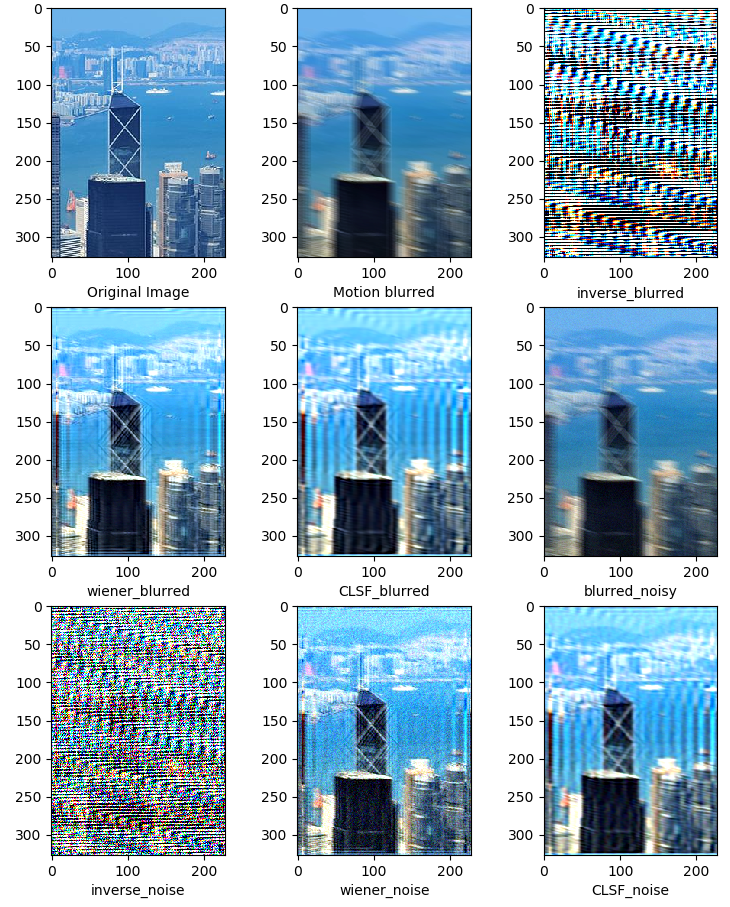展开全文• 该资源包括两部分代码，都是python实现，使用两种方式实现了模糊聚类，使用的数据集也在压缩包内，配置好环境可直接运行。模糊聚类
• 今天小编就为大家分享一篇关于Opencv+Python实现图像运动模糊和高斯模糊的示例，小编觉得内容挺不错的，现在分享给大家，具有很好的参考价值，需要的朋友一起跟随小编来看看吧
• 图像模糊处理的实现 包括有：均值模糊，高斯模糊，运动模糊 一.均值模糊 # 图像模糊处理 # 均值模糊 box blur import cv2 import numpy as np import matplotlib.pyplot as plt if __name__ == "__main__": image...

图像模糊处理的实现
包括有：均值模糊，高斯模糊，运动模糊
实质为使用滤波器算子对图像每个像素点进行卷积处理，改变像素值，实现图像的模糊化。

一.均值模糊

核心为使用均值滤波器。

# 图像模糊处理
# 均值模糊 box blur

import cv2
import numpy as np
import matplotlib.pyplot as plt

if __name__ == "__main__":

image = cv2.cvtColor(image, cv2.COLOR_BGR2GRAY)

# 此为均值模糊
# （30,1）为一维卷积核，指在x，y方向偏移多少位
dst1 = cv2.blur(image, (30, 1))

# 此为中值模糊，常用于去除椒盐噪声
dst2 = cv2.medianBlur(image, 15)

# 自定义卷积核，执行模糊操作，也可定义执行锐化操作
kernel = np.ones([5, 5], np.float32)/25
dst3 = cv2.filter2D(image, -1, kernel=kernel)

plt.subplot(2, 2, 1)
plt.imshow(image)
plt.axis('off')
plt.title('Offical')

plt.subplot(2, 2, 2)
plt.imshow(dst1)
plt.axis('off')
plt.title('Box blur')

plt.subplot(2, 2, 3)
plt.imshow(dst2)
plt.axis('off')
plt.title('median blur')

plt.subplot(2, 2, 4)
plt.imshow(dst3)
plt.axis('off')
plt.title('defined blur')

plt.show()

效果展示：实际上这里使用了均值滤波器、中值滤波器和一个自定义卷积核的滤波器来比较效果。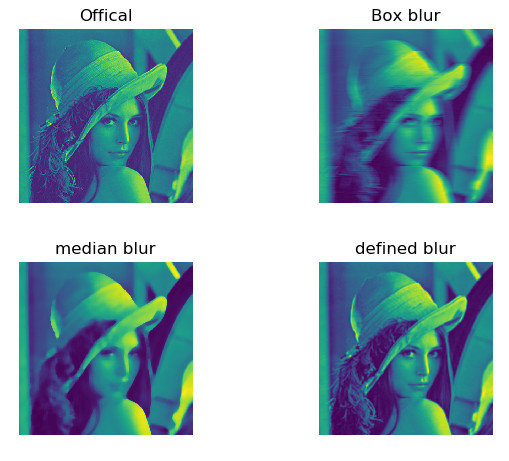二.高斯模糊

# 图像模糊处理
# 高斯模糊 gaussian blur
# 使用自编写高斯噪声及自编写高斯模糊函数与自带高斯函数作效果对比

import cv2
import numpy as np
import matplotlib.pyplot as plt

def clamp(pv):
if pv > 255:
return 255
if pv < 0:
return 0
else:
return pv

def gaussian_noise(image):        # 加高斯噪声
h, w, c = image.shape
for row in range(h):
for col in range(w):
s = np.random.normal(0, 20, 3)
b = image[row, col, 0]   # blue
g = image[row, col, 1]   # green
r = image[row, col, 2]   # red
image[row, col, 0] = clamp(b + s)
image[row, col, 1] = clamp(g + s)
image[row, col, 2] = clamp(r + s)
dst = cv2.GaussianBlur(image, (15, 15), 0)  # 高斯模糊
return dst, image

if __name__ == "__main__":

plt.subplot(2, 2, 1)
plt.imshow(src)
plt.axis('off')
plt.title('Offical')

output, noise = gaussian_noise(src)
cvdst = cv2.GaussianBlur(src, (15, 15), 0)   # 高斯模糊

plt.subplot(2, 2, 2)
plt.imshow(noise)
plt.axis('off')
plt.title('Gaussian Noise')

plt.subplot(2, 2, 3)
plt.imshow(output)
plt.axis('off')
plt.title('Gaussian Blur')

plt.subplot(2, 2, 4)
plt.imshow(cvdst)
plt.axis('off')
plt.title('defined blur by opencv')

plt.show()

结果展示：这里使用了自编写的高斯滤波器和opencv的高斯滤波器来对比效果。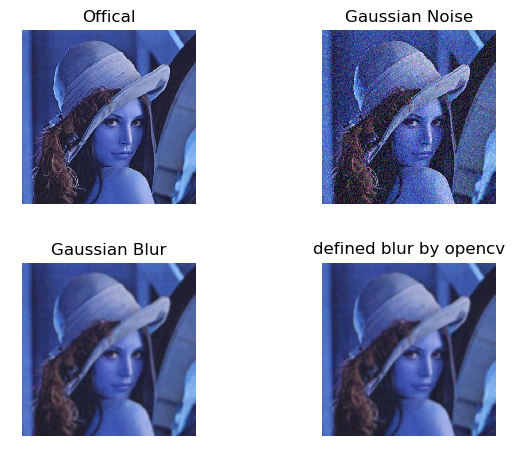三.运动模糊

使用自定义的卷积核进行卷积计算，实现运动模糊效果。

# 图像模糊处理
# 运动模糊，亦称动态模糊，motion blur
# 运动模糊：由于相机和物体之间的相对运动造成的模糊

import numpy as np
import cv2
import matplotlib.pyplot as plt

def motion_blur(image, degree=12, angle=45):

image = np.array(image)
# 这里生成任意角度的运动模糊kernel的矩阵， degree越大，模糊程度越高
M = cv2.getRotationMatrix2D((degree / 2, degree / 2), angle, 1)
motion_blur_kernel = np.diag(np.ones(degree))
motion_blur_kernel = cv2.warpAffine(motion_blur_kernel, M, (degree, degree))
motion_blur_kernel = motion_blur_kernel / degree
blurred = cv2.filter2D(image, -1, motion_blur_kernel)
# convert to uint8
cv2.normalize(blurred, blurred, 0, 255, cv2.NORM_MINMAX)
blurred = np.array(blurred, dtype=np.uint8)
return blurred

if __name__ == "__main__":

dst = motion_blur(img)

plt.subplot(1, 2, 1)
plt.imshow(img)
plt.axis('off')
plt.title('Offical')

plt.subplot(1, 2, 2)
plt.imshow(dst)
plt.axis('off')
plt.title('Motion blur')

plt.show()

结果展示：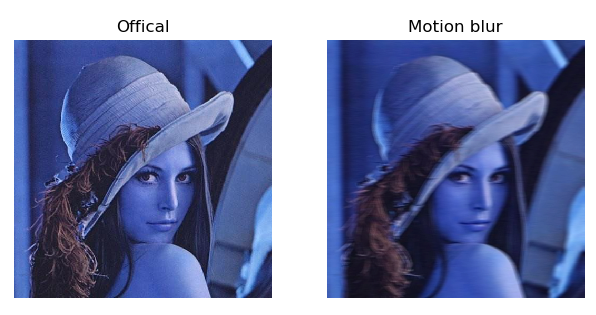展开全文opencv 图像处理
• python

千次阅读 2020-12-29 01:53:40
概述如何执行图像去模糊。通过FFT反卷积以及为什么这是个坏主意一种执行反卷积的替代方法但是首先，一些符号：我用I表示图像，用K表示卷积核。 I * K是图像I与内核K的卷积。 F (I)是图像I的(n维)傅立叶变换， F (K)...

不幸的是，您寻求解决的问题比您预期的要困难。 让我分四个部分进行说明。 第一部分假定您对傅立叶变换感到满意。

为什么您不能通过简单的反卷积来解决此问题。

概述如何执行图像去模糊。

通过FFT反卷积以及为什么这是个坏主意

一种执行反卷积的替代方法

但是首先，一些符号：

我用I表示图像，用K表示卷积核。 I * K是图像I与内核K的卷积。 F (I)是图像I的(n维)傅立叶变换， F (K)是卷积核K的傅立叶变换(也称为点扩展函数或PSF)。 同样， Fi是傅立叶逆变换。

为什么您不能通过简单的反卷积来解决此问题：

当你说我们能恢复模糊图像磅你是正确= I * K除以傅立叶变换K的傅立叶变换Ib的。 但是，镜头模糊不是卷积模糊操作。 这是一种改进的卷积模糊运算，其中模糊核K取决于与您拍摄的物体的距离。 因此，内核随像素变化。

您可能会认为这与图像无关，因为您已经在图像位置测量了正确的内核。 但是，情况可能并非如此，因为图像的较远部分会影响图像的较近部分。 解决此问题的一种方法是裁剪图像，以便仅可见纸。

为什么通过FFT解卷积是一个坏主意：

卷积定理指出I * K = Fi ( F (I) F (K)) 。 该定理导致一个合理的假设，即如果我们有一个图像， Ib = I * K被卷积核K模糊，那么我们可以通过计算I =( F (Ib)/ F (K))来恢复去模糊的图像。 。

在我们探讨为什么这不是一个好主意之前，我想对卷积定理的含义有一些直觉。 当我们将图像与内核卷积时，这与获取图像的频率分量并将其逐元素乘以内核的频率分量相同。

现在，让我解释一下为什么很难用FFT对图像进行卷积处理。 默认情况下，模糊会删除高频信息。 因此， K的高频必须接近零。 原因是I的高频信息在模糊时会丢失-因此， Ib的高频分量必须趋于零。 为此， K的高频分量也必须趋近于零。

由于K的高频分量几乎为零，因此我们发现，当我们对FFT解卷积时， Ib的高频分量被显着放大(因为我们几乎被零除)。 在无噪声的情况下这不是问题。

但是，在嘈杂的情况下，这是一个问题。 其原因是，按照定义，噪声就是高频信息。 因此，当我们尝试对Ib进行反卷积时，噪声几乎被无限放大。 这就是通过FFT进行反卷积不是一个好主意的原因。

此外，您需要考虑基于FFT的卷积算法如何处理边界条件。 通常，当我们对图像进行卷积时，分辨率会有所降低。 这是不必要的行为，因此我们引入了边界条件，这些条件指定了图像外部像素的像素值。 这样的边界条件的例子是

图像外部的像素与图像内部最近的像素具有相同的值

图像外部的像素具有恒定值(例如0)

该图像是周期信号的一部分，因此最上面一行上方的像素行等于最下面一行像素。

最终边界条件通常对一维信号有意义。 但是，对于图像来说，这毫无意义。 不幸的是，卷积定理规定使用周期性边界条件。

除此之外，似乎基于FFT的反演方法比迭代方法(例如，梯度下降和FISTA)对错误的内核更加敏感。

一种执行反卷积的替代方法

由于所有图像都嘈杂，因此看起来似乎已经失去了所有希望，而去卷积将增加噪点。 但是，事实并非如此，因为我们有迭代方法来执行去卷积。 首先让我向您展示最简单的迭代方法。

让|| I ||²是所有I像素的平方和。 解方程

Ib = I * K

关于I ，则相当于解决以下优化问题：

min L(I)= min || I * K-Ib ||²

关于我 。 这可以使用梯度下降来完成，因为L的梯度由

DL = Q *(I * K-Ib)

其中Q是通过转置K得到的内核(在信号处理文献中也称为匹配滤波器)。

因此，您可以获得以下将对图像进行模糊处理的迭代算法。

from scipy.ndimage import convolve

learning_rate = # You need to find this yourself, do a logarithmic line search. Small rate will always converge, but slowly. Start with 0.4 and divide by 2 every time it fails.

maxit = 100

def loss(image):

return np.sum(convolve(image, kernel) - blurred_image)

return convolve(convolve(image, kernel) - blurred_image)

deblurred = blurred_image.copy()

for _ in range(maxit):

上面的方法可能是最简单的迭代反卷积算法。 在实践中使用这些方法的方式是通过所谓的正则反卷积算法。 这些算法通过首先指定一个函数的工作，其测量噪声的图像中的量，例如TV(I)(I的总变化)。 然后在L(I)+ wTV(I)上执行优化过程。 如果您对此类算法感兴趣，建议阅读Amir Beck和Marc Teboulle撰写的FISTA论文。 这篇论文的数学运算量很大，但是您不需要了解其中的大部分内容，只需了解如何实现电视去模糊算法即可。

除了使用调节器外，我们还使用加速方法来使损失L(I)最小化。 内斯特罗夫加速梯度下降就是这样一个例子。 有关此类方法的信息，请参阅Emmanuel Candes的Brendan O'Donoghue的《自适应加速梯度方案的重新启动》。

概述如何执行图像去模糊。

裁剪图像，使一切与相机的距离相同

以与现在相同的方式查找卷积内核(首先在合成模糊图像上测试去卷积算法)

实施迭代方法来计算去甲壳素

对图像进行反卷积。

展开全文• 主要是深度学习模型进行图像增强，实现深度学习训练阶段做图像扩增，增加模型的泛化能力。
• 去除由于对焦，运动等造成的模糊图像，所以在构建数据集的时候考虑用opencv对清晰的图片进行处理获得模糊的图片从而进行训练。1) 运动模糊图像一般来说，运动模糊图像都是朝同一方向运动的，那么就可以利用cv2....
• python图像处理：基于canny的动态边缘模糊效果原理canny边缘检测代码 效果 流程如下:先选择canny的两个阈值，确定好需要进行模糊的边缘，再选择模糊程度 原理 canny边缘检测 参考链接 Canny边缘检测算法可以分为...算法 opencv 计算机视觉 python
• python实现图像模糊

千次阅读 2019-03-08 20:35:59
代码：高斯模糊 from PIL import Image, ImageFilter import numpy as np img = Image.open("./1.jpg").filter(ImageFilter.GaussianBlur) img.save("1_.jpg") 原图： ...
• 代码用于实现图像数据增强，对图片进行批量处理。包括图片旋转、翻转、模糊、增加噪声、亮度几种处理。运行需要安装python、opencv、numpy等。 使用时将图片统一放在img文件夹中，并将img文件夹和下载的py文件放在...
• super pix图像清晰化（超级分辨率）采用python语言编写程序实现
• Python图像聚类

2019-03-05 10:22:28
请结合本人该篇博客进行理解： https://blog.csdn.net/weixin_44344462/article/details/88169565
• 图像去模糊算法代码实践！

千次阅读 多人点赞 2021-09-25 00:24:53
目前GAN有很多应用，每个应用对应的论文和Pytorch代码可以参考下面的链接，其中也有GAN的代码，大家可以根据代码进一步理解GAN：https://github.com/eriklindernoren/PyTorch-GAN 2.图像去模糊算法：DeblurGANv2 ...算法 python 计算机视觉 机器学习 人工智能
• python处理模糊图像过滤

千次阅读 2019-04-17 13:22:50
最近在做人脸识别清洗样本的工作，发现经过人脸对齐后存在部分图片...图片越模糊，imageVar的值越小，图像模糊。 #-*-coding:utf-8-*- import sys #reload(sys) #sys.setdefaultencoding('utf-8') import os i...
• Project page] 2007-CVPR - 使用透明度的单幅图像运动去模糊。 [论文] 2008-CVPR - 使用锐边预测的 Psf 估计。 [Paper][Project page] 2008-TOG - 单张图像的高质量运动去模糊。 [Paper][Code & Project ...机器学习图像处理 特征提取
• 运动模糊： 由于相机和物体之间的相对运动造成的模糊，又称为动态模糊OpenCV+Python实现运动模糊，主要用到的函数是cv2.filter2D()：# coding: utf-8import numpy as npimport cv2def motion_blur(image, degree=12,...
• 目标检测，图像已经用labelimg标注好目标，想实现运动模糊，有没有哪位有实现的相关代码，谢谢了，50c</p>深度学习 python
• 欢迎关注“小白玩转Python”，发现更多 “有趣”引言在之前的文章中，我们讨论了边缘检测内核。在本文我们将讨论如何在图像上应用模糊与锐化内核，将这些内核应用到彩色图像上，同时保留核心...内核 计算机视觉 opencv python 人工智能
• 模糊操作是图像处理中最简单和常用的操作之一，该使用的操作之一原因就为了给图像预处理时减低噪声，基于数学的卷积操作均值模糊，函数 cv2.blur(image,(5,5))，这是一个平滑图片的函数，它将一个区域内所有点的灰度...
• 利用Laplacian变换进行图像模糊检测 核心代码： def blurryImgs(files,clear_img_list): count = 0 imgfile = files for img in files: if img.endswith('.jpg'): image = cv2.imread(img) gray = cv
• python实现了图像的混沌加密与解密，是参考某位大佬博客上面的用matlab实现了的加密解密算法...

python图像去模糊代码python 订阅